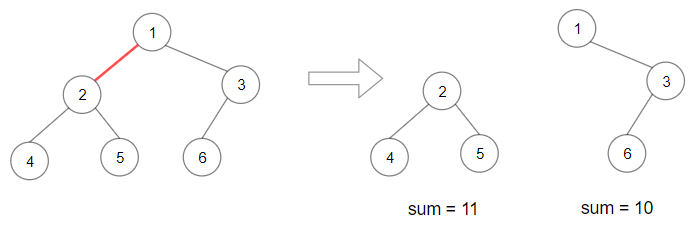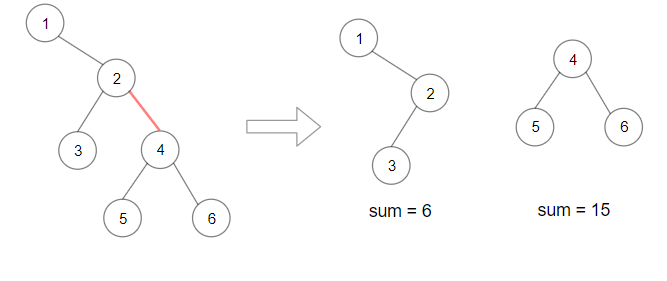Leetcode 1339：分裂二叉树的最大乘积（超详细的解法！！！）
in leetcode with 0 comment输入：root = [1,2,3,4,5,6]输入：root = [1,null,2,3,4,null,null,5,6]

输入：root = [2,3,9,10,7,8,6,5,4,11,1]

输入：root = [1,1]

• 每棵树最多有 50000 个节点，且至少有 2 个节点。
• 每个节点的值在 [1, 10000] 之间。

class Solution:
def maxProduct(self, root: TreeNode) -> int:
total = 0
def inOrder(node):
nonlocal total
if node:
inOrder(node.left)
total += node.val
inOrder(node.right)
inOrder(root)

mod, res = 10**9 + 7, 0
def dfs(node):
nonlocal res
if not node:
return 0

l, r = dfs(node.left), dfs(node.right)
res =  max(res, l * (total - l), r * (total - r))
return l + r + node.val

dfs(root)
return res % mod

class Solution:
def maxProduct(self, root: TreeNode) -> int:
total, mod, res = 0, 10**9 + 7, 0
def dfs(node):
nonlocal res
if not node:
return 0

l, r = dfs(node.left), dfs(node.right)
res =  max(res, l * (total - l), r * (total - r))
return l + r + node.val

total = dfs(root)
dfs(root)
return res % mod

reference:

https://leetcode.com/problems/maximum-product-of-splitted-binary-tree/discuss/496549/JavaC%2B%2BPython-Easy-and-Concise

「如果我的文章对你有很大帮助，那么不妨～！」Responses